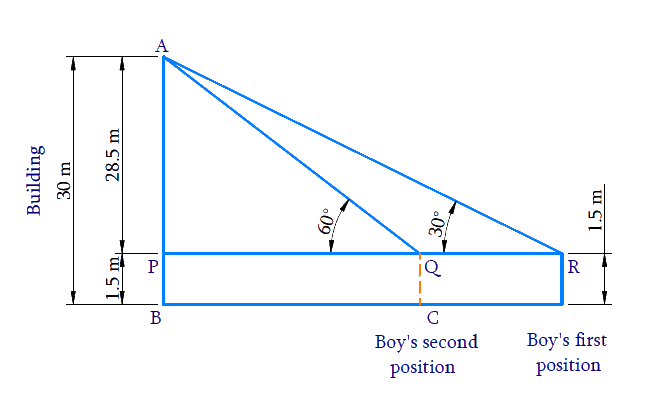# Ex.9.1 Q6 Some Applications of Trigonometry Solution - NCERT Maths Class 10

Go back to  'Ex.9.1'

## Question

A $$1.5\,\rm{m}$$ tall boy is standing at some distance from a $$30\,\rm{m}$$ tall building. The angle of elevation from his eyes to the top of the building increases from $$30^\circ$$ to $$60^\circ$$ as he walks towards the building. Find the distance he walked towards the building.

Video Solution
Some Applications Of Trigonometry
Ex 9.1 | Question 6

## Text Solution

What is Known?

(i) Height of the boy $$= 1.5\,{\rm{ m}}$$

(ii) Height of the building $$= 30\,{\rm {m}}$$

(iii) Angle of elevation from his eyes to the top of the building increases from $$30^\circ$$ to $$60^\circ$$ as he walks toward the building.

What is Unknown?

Distance, the boy walked towards the buildingReasoning:

Trigonometric ratio involving ($$AP, PR$$ and $$\angle R$$) and ($$AP, PQ$$  and $$\angle Q$$) is $$tan \,\theta$$

[Refer thet  diagram to visualise $$AP, PR$$ and $$PQ$$]

Distance walked towards the building $$RQ$$ $$= PR – PQ$$

Steps:

In $$\Delta \mathrm{APR}$$

\begin{align} \tan R &=\frac{A P}{P R} \\ \tan 30^{\circ} &=\frac{28.5}{P R} \\ \frac{1}{\sqrt{3}} &=\frac{28.5}{P R} \\ P R &=28.5 \times \sqrt{3} \mathrm{m} \end{align}

In $$\Delta \mathrm{APQ}$$

\begin{align}{\tan \mathrm{Q}}&={\frac{A P}{P Q}} \\ {\tan 60^{\circ}}&={\frac{28.5}{P Q}}\\ \sqrt{3} &=\frac{28.5}{P Q} \\ P Q &=\frac{28.5}{\sqrt{3}} \mathrm{m} \end{align}

Therefore,

\begin{align}PR - PQ &= 28.5\sqrt 3 - \frac{{28.5}}{{\sqrt 3 }}\\ &= 28.5\left( {\sqrt 3 - \frac{1}{{\sqrt 3 }}} \right)\\&= 28.5\left( {\frac{{3 - 1}}{{\sqrt 3 }}} \right)\\&= 28.5\left( {\frac{2}{{\sqrt 3 }}} \right)\\&= \frac{{57}}{{\sqrt 3 }}\\&= \frac{{57}}{{\sqrt 3 }} \times \frac{{\sqrt 3 }}{{\sqrt 3 }}\\\rm&= \frac{{57\sqrt 3 }}{3}\\&= 19\sqrt 3 {\rm{m}}\end{align}

Distance the boy walked towards the building is $$19 \sqrt{3} \mathrm{m}$$.

Learn from the best math teachers and top your exams

• Live one on one classroom and doubt clearing
• Practice worksheets in and after class for conceptual clarity
• Personalized curriculum to keep up with school